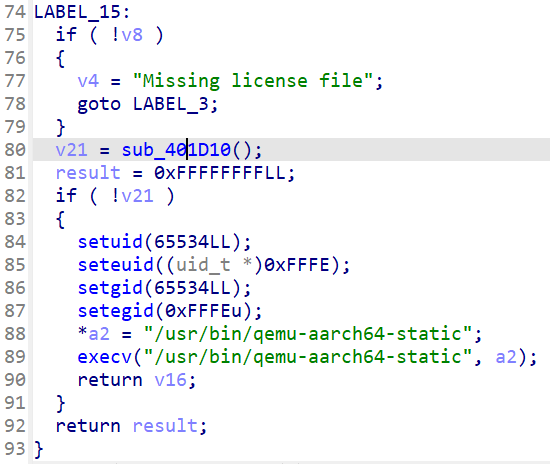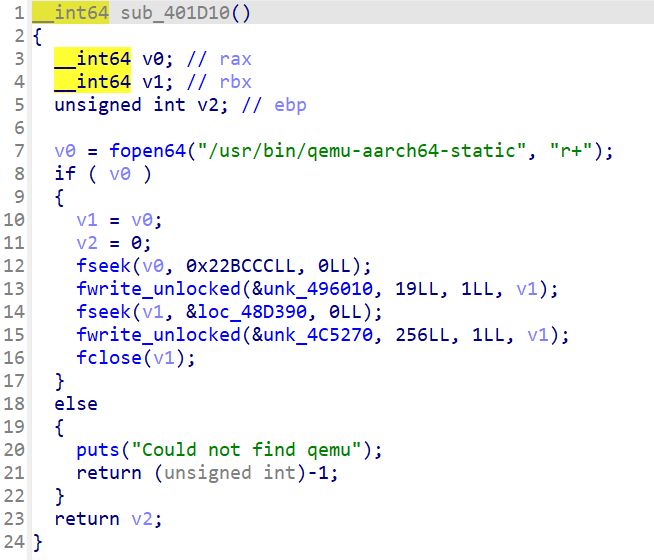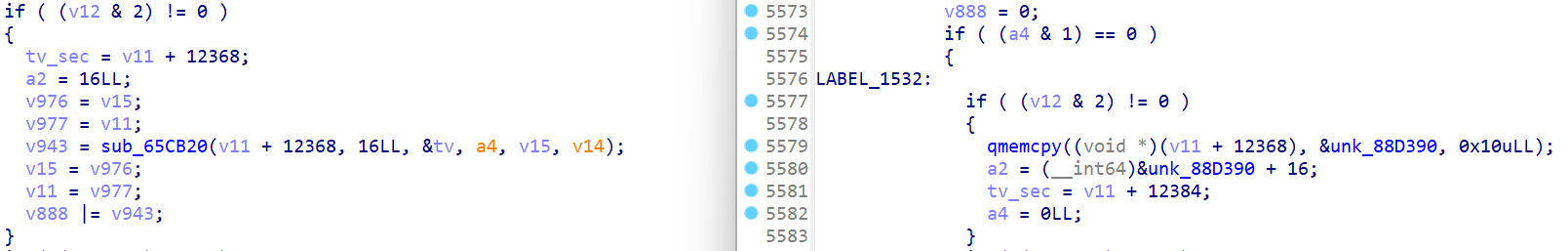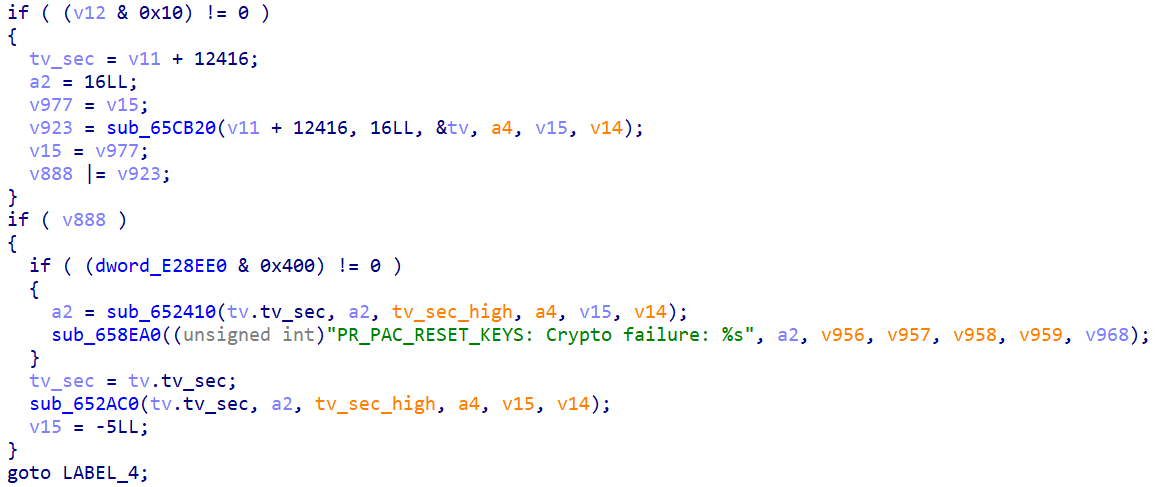# DEFCON CTF 2023 Qualifier Writeup

In the DEFCON CTF Qualifier this year, I mainly contributed in challenge opacity and blackbox. This post contains the writeup for them.

# opacity

In this challenge, we are given two ELFs init_drm and run_prog. We can use ./init_drm <program.license> ./run_prog <program.bin> to run some programs.

After some basic tests, we can find that a program only runs with correct license. The given triangle.bin will print a triangle, and password_for_flag.bin might give us the flag.

## init_drm

First, let's check init_drm.It does only one things: patch qemu with some code and the license file.

But what are these patched values? Let's take a look in this specific qemu-aarch64-static.Here is a comparison of them, the left is before patch, the right is after patch. The unk_88D390 points to the patched license.

In order to identify this part in the giant function, we could check the debugger symbols. However, I didn't do so.In the later part, we can find such a string, and it's easy to find the corresponding function at https://github.com/qemu/qemu/blob/f9baca549e44791be0dd98de15add3d8452a8af0/linux-user/syscall.c#L10775.

As a result, we know that the patched qemu will write the license to PAC keys when reset, instead of random keys.

## run_prog

The hard reverse process is mainly done by my teammates. Here are some key points:

• It uses union to store both one gate and a list of gate, which is very confuse when reversing.
• The gate execution is done by AUTIB1716. But this instruction is ignored by the decompiler of IDA. So we haven't point out how the gates are executed for a long time.
• Its execution is done by topological sorting in sub_2385D8. In sub_23A814, it initializes all nodes without any other affecting them. In sub_238388, it checks the affected nodes by one node.

We can find the PAC authentication code in https://github.com/qemu/qemu/blob/f9baca549e44791be0dd98de15add3d8452a8af0/target/arm/pauth_helper.c, and calculate the truth table for all gates:

#include<stdio.h>
#include<stdint.h>
#include<assert.h>
#include<string.h>
#include<stdlib.h>
(((~0ULL) >> (64 - (length))) << (shift))

static inline uint64_t deposit64(uint64_t value, int start, int length,
uint64_t fieldval)
{
assert(start >= 0 && length > 0 && length <= 64 - start);
mask = (~0ULL >> (64 - length)) << start;
}

static inline int64_t sextract64(uint64_t value, int start, int length)
{
assert(start >= 0 && length > 0 && length <= 64 - start);
/* Note that this implementation relies on right shift of signed
* integers being an arithmetic shift.
*/
return ((int64_t)(value << (64 - length - start))) >> (64 - length);
}

static inline uint32_t extract32(uint32_t value, int start, int length)
{
assert(start >= 0 && length > 0 && length <= 32 - start);
return (value >> start) & (~0U >> (32 - length));
}

static int rot_cell(int cell, int n)
{
/* 4-bit rotate left by n.  */
cell |= cell << 4;
return extract32(cell, 4 - n, 4);
}

static inline uint64_t extract64(uint64_t value, int start, int length)
{
assert(start >= 0 && length > 0 && length <= 64 - start);
return (value >> start) & (~0ULL >> (64 - length));
}

static uint64_t pac_cell_shuffle(uint64_t i)
{
uint64_t o = 0;

o |= extract64(i, 52, 4);
o |= extract64(i, 24, 4) << 4;
o |= extract64(i, 44, 4) << 8;
o |= extract64(i,  0, 4) << 12;

o |= extract64(i, 28, 4) << 16;
o |= extract64(i, 48, 4) << 20;
o |= extract64(i,  4, 4) << 24;
o |= extract64(i, 40, 4) << 28;

o |= extract64(i, 32, 4) << 32;
o |= extract64(i, 12, 4) << 36;
o |= extract64(i, 56, 4) << 40;
o |= extract64(i, 20, 4) << 44;

o |= extract64(i,  8, 4) << 48;
o |= extract64(i, 36, 4) << 52;
o |= extract64(i, 16, 4) << 56;
o |= extract64(i, 60, 4) << 60;

return o;
}

static uint64_t pac_cell_inv_shuffle(uint64_t i)
{
uint64_t o = 0;

o |= extract64(i, 12, 4);
o |= extract64(i, 24, 4) << 4;
o |= extract64(i, 48, 4) << 8;
o |= extract64(i, 36, 4) << 12;

o |= extract64(i, 56, 4) << 16;
o |= extract64(i, 44, 4) << 20;
o |= extract64(i,  4, 4) << 24;
o |= extract64(i, 16, 4) << 28;

o |= i & MAKE_64BIT_MASK(32, 4);
o |= extract64(i, 52, 4) << 36;
o |= extract64(i, 28, 4) << 40;
o |= extract64(i,  8, 4) << 44;

o |= extract64(i, 20, 4) << 48;
o |= extract64(i,  0, 4) << 52;
o |= extract64(i, 40, 4) << 56;
o |= i & MAKE_64BIT_MASK(60, 4);

return o;
}

static uint64_t pac_sub(uint64_t i)
{
static const uint8_t sub = {
0xb, 0x6, 0x8, 0xf, 0xc, 0x0, 0x9, 0xe,
0x3, 0x7, 0x4, 0x5, 0xd, 0x2, 0x1, 0xa,
};
uint64_t o = 0;
int b;

for (b = 0; b < 64; b += 4) {
o |= (uint64_t)sub[(i >> b) & 0xf] << b;
}
return o;
}

static uint64_t pac_inv_sub(uint64_t i)
{
static const uint8_t inv_sub = {
0x5, 0xe, 0xd, 0x8, 0xa, 0xb, 0x1, 0x9,
0x2, 0x6, 0xf, 0x0, 0x4, 0xc, 0x7, 0x3,
};
uint64_t o = 0;
int b;

for (b = 0; b < 64; b += 4) {
o |= (uint64_t)inv_sub[(i >> b) & 0xf] << b;
}
return o;
}

static uint64_t pac_mult(uint64_t i)
{
uint64_t o = 0;
int b;

for (b = 0; b < 4 * 4; b += 4) {
int i0, i4, i8, ic, t0, t1, t2, t3;

i0 = extract64(i, b, 4);
i4 = extract64(i, b + 4 * 4, 4);
i8 = extract64(i, b + 8 * 4, 4);
ic = extract64(i, b + 12 * 4, 4);

t0 = rot_cell(i8, 1) ^ rot_cell(i4, 2) ^ rot_cell(i0, 1);
t1 = rot_cell(ic, 1) ^ rot_cell(i4, 1) ^ rot_cell(i0, 2);
t2 = rot_cell(ic, 2) ^ rot_cell(i8, 1) ^ rot_cell(i0, 1);
t3 = rot_cell(ic, 1) ^ rot_cell(i8, 2) ^ rot_cell(i4, 1);

o |= (uint64_t)t3 << b;
o |= (uint64_t)t2 << (b + 4 * 4);
o |= (uint64_t)t1 << (b + 8 * 4);
o |= (uint64_t)t0 << (b + 12 * 4);
}
return o;
}

static uint64_t tweak_cell_rot(uint64_t cell)
{
return (cell >> 1) | (((cell ^ (cell >> 1)) & 1) << 3);
}

static uint64_t tweak_shuffle(uint64_t i)
{
uint64_t o = 0;

o |= extract64(i, 16, 4) << 0;
o |= extract64(i, 20, 4) << 4;
o |= tweak_cell_rot(extract64(i, 24, 4)) << 8;
o |= extract64(i, 28, 4) << 12;

o |= tweak_cell_rot(extract64(i, 44, 4)) << 16;
o |= extract64(i,  8, 4) << 20;
o |= extract64(i, 12, 4) << 24;
o |= tweak_cell_rot(extract64(i, 32, 4)) << 28;

o |= extract64(i, 48, 4) << 32;
o |= extract64(i, 52, 4) << 36;
o |= extract64(i, 56, 4) << 40;
o |= tweak_cell_rot(extract64(i, 60, 4)) << 44;

o |= tweak_cell_rot(extract64(i,  0, 4)) << 48;
o |= extract64(i,  4, 4) << 52;
o |= tweak_cell_rot(extract64(i, 40, 4)) << 56;
o |= tweak_cell_rot(extract64(i, 36, 4)) << 60;

return o;
}

static uint64_t tweak_cell_inv_rot(uint64_t cell)
{
return ((cell << 1) & 0xf) | ((cell & 1) ^ (cell >> 3));
}

static uint64_t tweak_inv_shuffle(uint64_t i)
{
uint64_t o = 0;

o |= tweak_cell_inv_rot(extract64(i, 48, 4));
o |= extract64(i, 52, 4) << 4;
o |= extract64(i, 20, 4) << 8;
o |= extract64(i, 24, 4) << 12;

o |= extract64(i,  0, 4) << 16;
o |= extract64(i,  4, 4) << 20;
o |= tweak_cell_inv_rot(extract64(i,  8, 4)) << 24;
o |= extract64(i, 12, 4) << 28;

o |= tweak_cell_inv_rot(extract64(i, 28, 4)) << 32;
o |= tweak_cell_inv_rot(extract64(i, 60, 4)) << 36;
o |= tweak_cell_inv_rot(extract64(i, 56, 4)) << 40;
o |= tweak_cell_inv_rot(extract64(i, 16, 4)) << 44;

o |= extract64(i, 32, 4) << 48;
o |= extract64(i, 36, 4) << 52;
o |= extract64(i, 40, 4) << 56;
o |= tweak_cell_inv_rot(extract64(i, 44, 4)) << 60;

return o;
}

static uint64_t pauth_computepac_architected(uint64_t data, uint64_t modifier,
uint64_t key0, uint64_t key1)
{
static const uint64_t RC = {
0x0000000000000000ull,
0x13198A2E03707344ull,
0xA4093822299F31D0ull,
0x082EFA98EC4E6C89ull,
0x452821E638D01377ull,
};
const uint64_t alpha = 0xC0AC29B7C97C50DDull;
/*
* Note that in the ARM pseudocode, key0 contains bits <127:64>
* and key1 contains bits <63:0> of the 128-bit key.
*/
uint64_t workingval, runningmod, roundkey, modk0;
int i;

modk0 = (key0 << 63) | ((key0 >> 1) ^ (key0 >> 63));
runningmod = modifier;
workingval = data ^ key0;

for (i = 0; i <= 4; ++i) {
roundkey = key1 ^ runningmod;
workingval ^= roundkey;
workingval ^= RC[i];
if (i > 0) {
workingval = pac_cell_shuffle(workingval);
workingval = pac_mult(workingval);
}
workingval = pac_sub(workingval);
runningmod = tweak_shuffle(runningmod);
}
roundkey = modk0 ^ runningmod;
workingval ^= roundkey;
workingval = pac_cell_shuffle(workingval);
workingval = pac_mult(workingval);
workingval = pac_sub(workingval);
workingval = pac_cell_shuffle(workingval);
workingval = pac_mult(workingval);
workingval ^= key1;
workingval = pac_cell_inv_shuffle(workingval);
workingval = pac_inv_sub(workingval);
workingval = pac_mult(workingval);
workingval = pac_cell_inv_shuffle(workingval);
workingval ^= key0;
workingval ^= runningmod;
for (i = 0; i <= 4; ++i) {
workingval = pac_inv_sub(workingval);
if (i < 4) {
workingval = pac_mult(workingval);
workingval = pac_cell_inv_shuffle(workingval);
}
runningmod = tweak_inv_shuffle(runningmod);
roundkey = key1 ^ runningmod;
workingval ^= RC[4 - i];
workingval ^= roundkey;
workingval ^= alpha;
}
workingval ^= modk0;

return workingval;
}

uint64_t test(uint32_t a,uint32_t b)
{
return pauth_computepac_architected(a,b,0xcf1b86873198a7bdull,0xd6c912742cd0b7f9ull)>>48&0x7f;
}

uint32_t gates;

int main(int argc, char** argv) {
FILE*f=fopen("dist/data/triangle.bin","rb");
fseek(f,0xb0,SEEK_SET);
assert(ftell(f)==18796);
fclose(f);
freopen("table.txt","w",stdout);
for(int i=0;i<665;i++)
{
if((gates[i]&0xff)==0)
{
int x=(gates[i]-0x4040000)/28,y=(gates[i]-0x4040000)/28;
uint64_t x1=gates[x],x0=gates[x],y1=gates[y],y0=gates[y],
tweak=gates[i]>>8&255,check=gates[i]>>16;
printf("%d %d %d %d %d\n",
i,
check==test(x0,y0+tweak),
check==test(x0,y1+tweak),
check==test(x1,y0+tweak),
check==test(x1,y1+tweak));
}
else
{
assert(gates[i]==0||gates[i]==0);
}
}
}


With the reversing done, we can try to find the flag.

In the given files, there are no license for password_for_flag.bin, so we can't directly execute it.

However, we can notice that the structure of password_for_flag.bin is exactly the same as triangle.bin, so we transplants the PAC values from triangle to password_for_flag.bin, and it seems worked.

Finally, I wrote a symbolic simulator using z3, and it produced some passwords. My teammates have different solutions, such as reversing the gates manually.

import sys, struct
import z3

def gate(x):
if x == 0:
return -1
else:
assert (x - 0x4040000) % 28 == 0
return (x - 0x4040000) // 28

h = s[:0xb0]

memory = h[0x90:]
ha = struct.unpack('i' * 36, h[:0x90])
iter_count = ha
ha = list(map(lambda x: (x - 0x4040000) // 28, ha))
is_out = ha
out_regs = ha[3:11]
is_in = ha
in_regs = ha[12:20]
is_exit = ha
is_error = ha
is_giveflag = ha

s = s[0xb0:]
gates = []
while s:
tp, tweak, check, lgate, rgate, lconst, rconst, oconst, dep = struct.unpack('<BBHIIIIII', s[:28])
gates.append((tp & 7, tweak, check, gate(lgate), gate(rgate), lconst, rconst, oconst, dep))
assert dep == 0
s = s[28:]

val = [-1] * len(gates)
xo =  * len(gates)

for i, (tp, tweak, check, lgate, rgate, lconst, rconst, oconst, dep) in enumerate(gates):
assert dep == 0
if tp == 5 or tp == 3:
assert oconst == lconst or oconst == rconst
val[i] = int(oconst == lconst)
elif tp == 0:
assert gates[lgate] != 4
assert gates[rgate] != 4
elif tp == 4:
xo[i] = int(gates[lgate] != lconst)
pass
else:
assert False

tt = [None] * len(gates)
i, *o = map(int, line.split())
tt[i] = o

q = []
c =  * len(gates)
for i in range(len(gates)):
if gates[i] == 0:
c[gates[i]] += 1
c[gates[i]] += 1
for i in range(len(gates)):
if gates[i] == 0 and c[i] == 0:
q.append(i)
i = 0
while i < len(q):
x = q[i]
i += 1
c[gates[x]] -= 1
c[gates[x]] -= 1
if gates[gates[x]] == 0 and c[gates[x]] == 0:
q.append(gates[x])
if gates[gates[x]] == 0 and c[gates[x]] == 0:
q.append(gates[x])
for i in range(len(gates)):
assert c[i] == 0
q = q[::-1]

r = 0
for i in range(len(arr)):
r |= val[arr[i]] << i
return r

def write_val(val, arr, v):
for i in range(len(arr)):
val[arr[i]] = v >> i & 1

for i in range(len(gates)):
if gates[i] == 0:
if tt[i]:
tt[i] = lambda x, y: (1 ^ x) & (1 ^ y)
elif tt[i]:
tt[i] = lambda x, y: (1 ^ x) & y
elif tt[i]:
tt[i] = lambda x, y: x & (1 ^ y)
elif tt[i]:
tt[i] = lambda x, y: x & y

val = [z3.BitVecVal(x, 8)for x in val]
flag = [z3.BitVec('x' + str(i), 8)for i in range(21)]
in_p = 0
some_flag = 0
solver = z3.Solver()
for x in flag:

def get_unique_value(x):
solver.push()
assert solver.check() == z3.sat
m = solver.model()
t = m.eval(x).as_long()
assert solver.check() == z3.unsat
solver.pop()
return t

for it in range(iter_count):
print(it)
for i in q:
l, r = gates[i][3:5]
val[i] = 1 ^ tt[i](val[l], val[r])
for i in range(len(gates)):
if gates[i] == 4 and gates[i] != -1:
val[i] = xo[i] ^ val[gates[i]]
for i in range(len(gates)):
if (gates[i] == 3 or gates[i] == 5) and gates[i] != -1:
val[i] = val[gates[i]]
for i in range(len(gates)):
val[i] = z3.simplify(val[i])
if it + 1 < iter_count:
if get_unique_value(val[is_in]):
write_val(val, in_regs, flag[in_p])
in_p += 1

solver.push()
solver.check()
m = solver.model()
t = m.eval(memptr).as_long()
v = z3.BitVecVal(memory[t], 8)
while solver.check() == z3.sat:
m = solver.model()
t = m.eval(memptr).as_long()
v = z3.If(memptr == t, z3.BitVecVal(memory[t], 8), v)
solver.pop()

some_flag |= val[is_giveflag]

s2 = z3.Solver()
for x in flag:
if s2.check() == z3.sat:
m = s2.model()
print(bytes([m.eval(x).as_long()for x in flag]))


# blackbox

We can send some code to execute in a VM, but we don't know anything about it.

Here's a timeline for solving it:

• A length should be given first as raw bytes. (Guess: 4 byte little endian)
• Write a script to manually test, with the ability to start over.
• 0070 -> A: 65535 B: 0 C: 0 D: 0: PC: 2.
• 9000 -> A: 16 B: 0 C: 0 D: 0: PC: 2.
• Try 90 + another byte, find 9008 and 9009 changed PC and SP, respectively. Also, 9010 seems to subtract from A.
• 90 is imm+80.
• Identified that length is given by 2 bytes, and it's instruction count instead of byte count.
• 0001 -> add B, A
• Instruction format: {1, imm[7:0]}, {opcode, reg} or {0, b'000 (?), reg}, {opcode, reg}
• Opcodes: 0 -> add, 1 -> sub, 2 -> load, 3 -> store, 4 -> and, 5 -> or, 6 -> xor, 7 -> neg, 10 -> jmp imm, 11 -> jmp pc+imm, 13 -> push, 14 -> pop.
• Guess: 12 -> conditional jump, 15 -> syscall
• Try to execute syscall(5), it requires a file descriptor. And syscall(4) says Error: Unable to open file.
• Open flag.
• Guess syscall 5 is read. Successfully read from flag. Use the printed registers to fetch 4 bytes of flag each time.